# A family of newforms

## Transcription

A family of newforms
```Commentationes in honorem
Olli Lehto
Annales Academire Scientiarum Fennicre
Series A. I. Mathematica
Volumen 10, 1985, 461-467
LX annos
nato
A FAMILY OF NEWFORMS
R. A. RANKIN
1. Introduction
Bounds for sums of powers of cusp form coefrcients were recently obtained
in tlOl. Subsequently, Moreno and Shahidi  have shown that, in the case of
Ramanujan's function z(n), more precise estimates can be obtained for the fourth
power. They have established that the Dirichlet series
)i=rra(n)n-"
has a double pole
at s:1312
(1.1)
ås
and so have deduced that
Z"=*[email protected])-Axlogx
[email protected]
where A is a positive constant and
(1.2)
to(n): t(n)n-Itz.
This improves the estimates
(1.3)
x<<
Zn=*[email protected])..xlogsx
found in . Presumably, asymptotic results of the form (1.1) hold also for fourth
powers of other newform coefficients not of complex multiplication type.
It is the purpose of the present paper to study an infinite family of newforms
of different weights for which precise asymptotic formulae can be found for every
sum of even powers, and which do not obey an asympiotic formula of the form (1.1)
for the fourth power sum of the coefficients. As indicated at the end of  these
forms are associated with Grössencharacters belonging to imaginary quadratic fields
and, for illustration, we take the simplest case, where the field is the field of Gaussian
integers
K:QQ\.
(€K
For each
(1.4)
we put
x(0
- r(€)(,
xoG)- xc)ll€l.
Here e(\$ is a Dirichlet character modulo the ideal (4) for which e(i): -1. For
such a character we must have e(l +2i): tl and, for definiteness, we take
(1.5)
doi:10.5186/aasfm.1985.1051
r(1 + 2i)
: - 1.
R. A. RaNrrN
462
Then, for each r€N,
fi
the ideal (4). Note that
e,
is a Grössencharacter for the field K and is associated with
when restricted to Z, is the non-principal character modulo 4.
Put
(1.6)
and observe
a,(n): I Ztel,=,t'G), a,(n): a,(n)n-'tz,
that, because of the properties of e, we have a,(1):a,(l):1. Let
(1.7)
g,(z)
:
)i=ra,(n)e2"in" (Imz >
0).
Then g, is a cusp form of weight r*l and level64, as noted
Our object is to obtain estimates for the sums
(1.8)
for each
A(F,r; x):
B>0 and r€N as [email protected] In
Theorem
C(N,
r)
l.
in  (Beispiel
3).
Zn=,1a,(n)lzo
particular, we shall prove
For any positbe integers
N
and
r
there exists a positiue number
such that
(1.9)
A(N,
r; x)-C(N, r)x (log x)o',
gg 16+a, where
(1.10)
*:
(t1,
To compare this result with (1.1) take
t)-'.
N:2
and note
that öz:2.
. Definitions
For each prime p=l (mod 4\,take any fixed (o€K such that l(olz:p. Then
every ( of normp is of the form
(:Eoin or (:€o(-i)",
where
v:0,1,2,3
xG\:e(to)to
Q.D
and the bar denotes the complex conjugate.
or its conjugate, and so
q,b):2Re{e(()(o/l(ol}': 2cosror
say, where we may assume
that 0=0r<n.
Accordingly, for any prime p, we have
(2.2)
a,(p)
:
where
(2'3)
rr0o
t,,r: l+n
2 cos 0,.o,
if p:1(mod4),
otherwise.
It
follows that
463
A family of newforms
Finally,let
(2.4')
(2.5)
Y"(s)
:
(l-Zp-"
[o:11moda)
cos
f) : Z]rlu,(n)lzf
G'(s,
vOr+p-2)-1,
n-",
the product and series being absolutely convergent for o:Res>1. We denote by
D the set of Dirichlet series holomorphic and nonzero for o>1.
3. Some lemmas
Lemma 3.1. For each r(N,u, is a multiplicatiue function. Moreouer, for any
prime
p
ntd positfue integer v
a,(pn
+t)
:
a,(p)
q(P') -
e(P)
a,(P'-t).
This may be proved exactly as in . It may be remarked that a,(n) is identical
with Gtaisher's function x,(n), which he defined  by
(3.1)
x,@)
_ ]_ z (_
1)("+ b- L)tz (a
+ ib)\, ,
the summation being taken over all rational integers a, å for which
a24b2:n, a odd, b even.
and 8 Glaisher used these functions in his work on the representations of
a number as a sum of l0 and 18 squares, respectively, and proved their multiplicative
properties. In earlier papers he used a slightly different notation that allowed ;9,(n)
to be nonzero for everi n.
From Lemma 3.1 and earlier remarks we deduce
For
r:4
Lemma 3.2.
character {+r.
The
fwtction g,(z) is a newform of weight
r*1,
leuel 64 and
We note that in some ways it is more natural to consider StrQ):S,kl8)' We
can then show that gf has level 8, weight r*1, character d+1 and divisor t:1,
in the notation of . Moreover, g! can be expressed in terms of derivatives of the
simple theta functions' If we write r2:9r'9r'9a, we find easily from the definition
>0,
(3.1) that, for
any r:?-rn* 1 with
(3.2)
s*,*,(z):ffiztr=,('";t)t-t)'nl"
while,
rn
e)*o-D(z),
for r:2rn>0,
(3.3)
et^Q)
:
ffi
zf =,('il) (- t;"s5'r (z)e\$n-r't (27.
That the expressions on the right-hand sides of (3.2) and (3.3) are in fact cusp
forms of weight r* I can be deduced by the methods developed in . Alternatively,
R. A. RaNrrN
464
at any rate when r is divisible by 4, we can deduce that g! is a modular form, since
in (3.1) (a-lib\ is a spherical harmonic polynomial; see Il].
Lemma 3.3. For each r€N, Yz,€D. Moreouer,for o>-1,
(3.4)
G,(s,l):
((s)Y2,G)ärG)
and
(3.5)
where H1 and
If p=t(modg(1-p-)-t: ((s)Ilr(s),
H, belong to D.
Proof. From Lemma 3 of  we deduce that G,(s,1)/((s)eO and also that
(3.4) holds with
:
flr(s)
(1-2-")Z(s,
which is clearly holomorphic and nonzero
e)
fi e=t(1-p-r),
for o> 1;
here and later we omit (mod 4)
from under product signs, for convenience.
The last part follows, since
JJ
p=t(l- p-')-'
:
II p=-L(l- p-'")L(s, e)z(s, e2).
Here the usual Z-function notation is used.
Lemma 3.4. Forfixed fl-O and r(N,
2zf
-L
lcos
|l'P
:
cp+ Zi=rrp(r)
cos
2n0
(0€ R),
where
2'8:!(f + tl2)
c6 -p
lir\$+D '
(3.6)
.
the Fourier cosine series being unifurmly conuergent on
for n=p and
(3.?)
R. If
P€N, then cp(n):O
"r:('u;t).
Proof. The function lcos 0l2f has period z and is continuous and of bounded
variation on [0, z].
4. Proofs of main results
From (2.2), (2.3) and Lemma 3.1 we deduce that
a,(p"):
and that
W
for p:1 (mod4), v€N,
ar(p'n): r, ar(p'n-t) : 0 for p: -1(mod 4), v€N.
A family of newforms
46s
Accordingly, for a1y B>O and o>1,
(4.1)
where,
for
p:l
(4.2)
and,
f) : II p,zG,(s, f , p),
c,(s,
(mod 4),
G,(s,
for p=
(4.3)
-l
f,p):
sin
1+i2cosr 0olzl p-"*)?|
tv=21
(v+!)rOe
sin
r0,
l'u
p-""
I
(mod 4),
p):
G,(s, F,
(1-P-21-t.
We are now in a position to prove Theorem l. We take a fixed
apply Leinma 3.4, so that we have, omitting the suffix p,
:
*
f :N€N
and
2l=, c (n) cos 2nr0 o.
It follows, on using Lemma 2 of  and (4.1-3), that
G, (s, f) : If p=
- p -2"1 -t II n=, (I - n-)-'" Il=r{Y2*(s)ft"r fI, (s)
12 cos r0 ,lqN
-t(1
Zc
:
2
("(s)äa(s),
by Lemma 3.3, with H"and H4tnD. Theorem
I now follows from the Delange-
Ikehara Theorem (see Lemma 4 of ), since
,:
12N-lt
"n: [ .lr ),
by Lemma 3.4.
Theorem 2. For any positiue e and p there exist positiae numbers A,, B,
depending only on r, e and B such that, for all sfficiently large x,
A,x(logx)öt8)-e = A(F'
where
ö(P):2u-1.
r; x) = B'x(lo9x)ö(6r+"'
Proof. Take any positive e and B. By Lemma 3.4 we may choose a positive
integer Ndepending on e and B such that
r0l2|-gr-=u_rcp(n)cos2nrll = e
for all real?.Take a prime p:l (mod 4). We then find that G,(s, f,p), regarded
as a power series in p-", is majorized by
l2zn-r lcos
GI
@,
f , p)
:
t-12{c+e+Zl=rc(n)cos2nr0o}n-"+Zlr(v+l1zn o-'"
and 'minorized' by
:
t+2{c - e+ fl^ c(n) cos 2nr0 o} P-".
Similar arguments to those used to prove Theorem I now show that G,(s, f) is
majorized by ("*'(s)I/u(s) and minorized by ("-"(s)äu(s), where Hu and Hu
belong to D. On applying the Delange-Ikehara theorem to these last two Dirichlet
G,- (s, f ,
series we complete the
p)
proof of Theorem 2. It is tempting to conjecture that Theorem
R. A. RaNrrN
466
2 can be replaced by the asymptotic result
g.q
A(f , r; x)-C76(lsg)c)a{F).
In this connexion it may be noted that,
replaced by the equivalent result:
for non-integral B, Theorem 2 can be
A(f , r; x) : x (log x1otO+orrr,
which is also derivable from a general theorem of Wirsing , by using the facto
proved by Hecke tzl,that,for p: I (mod 4),the angles 0n are uniformly distributed
in [0, z]. To remove the term o(1) from the exponent and introduce a constant
factor on the right of (4.5) would, however, require more information about the
behaviour ofthe sum
2 r=',o=' lcos r0rl2P
than is immediately deducible from this fact.
(4.5)
5. Concluding remarks
for
l. In [l0] upper (for B>1) and lower (for 0<p=1)
general newforms
Itisclear
bounds were obtained
of any level. The corresponding logarithmic exponent
y : y(p) : 22(0-L) -1.
that y(f)>ö(f) for B>l and y(D=6(f) for 0<B<1.
y(2):3, 6(2):2,
was
In particular,
as already remarkedn and
't(Il2):
-112, ö(Ll2): (2ln)-1.
be applied in conjunction with the result (1.1) of
2. The methods of  can
Moreno and Shahidi to replace the upper bound given in  by
(5.1)
Z,=*l"o(n)l2p << x (log x)c(p) (f = 2),
where
(5.2)
sff)
:
22P-s-L
-
y(f)
-
22P-2-1.
For this purpose one requires the inequality
l2cos|lzf < 2zF cosa g : 2zf -t(3*4cos20*cos40).
In the range l<B<.2, Hölder's inequality gives (5.1) with
(5.3)
(5.4)
a\$): f -r.
3. It is possible that the definitions (1.6) and (3.1) could be used to derive directly, without reference to modular form theory, the results obtained in this paper,
but my attempts to do so have been unsuccessful. However, it may be of interest to
remark that Titchmarsh's  two-dimensional version of van der Corput's method
can be directly used to show that
(5.5)
Zn*,a*(n) = O(*+"+"7,
467
A family of newforms
where oc:27182. This is an improvement on the best known bound for cusp form
coefficients of weight I *4r, namelY
O
(5.6)
(x"
+1/3
*').
No doubt the index a can be decreased slightly.
4. Similar methods can be applied to cusp forms associated with other imaginary quadratic fields and also, for example, to the field Q(r) for the other choice
of Dirichlet character in which (1.5) is replaced by e(l *2i\:1.
References
[l]
Gu,rsnrn, J. W. L.: On elliptic-function expansions in which the coefncients are powers of the
complex numbers having z as norm. - Quart. J. Pure Appl. Math. 39, 1908, 266300.
 Hrcrn, E.: Eine neue Art von Zetafunktion und ihre Beziehungen zur Verteilung der Primzahlen.
II. - Math. 2.6, 1920, 11-51; Mathematische Werke, Vandenhoek & Ruprecht,
Göttingen, 19 59, 249-289.
 Hncrr, E.: Uber Dirichlet-Reihen mit Funktionalgleichung und ihre Nullstellen auf der Mittelgeraden. - s.-B. Math.-Nat. Abt. Beyer. Akad. wiss. 1937,73-95; Mathematische
Werke, Vandenhoek
& Ruprecht, Göttingen,
1959' 708-730.
 MonrNo, c. J., and F. Snnrupr: The fourth moment of the Ramanujan z-function.
- Math.
Ann. 266, 1983, 233---239.
A.: Contributions to the theory of Ramanujan's function c(n) and similar arithme'
R.
RnNrrN,

tical functions. I. The order of the Fourier coefficients of integral modular forms. Proc. Cambridge Philos. Soc. 35, 1939, 357-372.
A.: A certain class of multiplicative functions. - Duke Math. J.13,1946,281-306'
given form.  ReNrrN, R. A.: The construction of automorphic forms from the derivatives of a
103-116.
20,
1956,
J. Indian Math. Soc.
 RrNrrx,R.A.:An,(2-resultforthecoemcientsof cusp forms. - Math. Ann.2O3,1973,2392so.
Cambridge RaNrrN, R. A,: Modular forms and functions. - Cambridge University Press,
 R.nNrrN, R.
London-:-New York-Melboune, 1977'
I10] RnxrrN, R. A.: Sums of powers of cusp form coefficients. - Math. Ann. 263, 1983, 227-136.
[ll] ScnonNrsnnc, B.: Das Verhalten von mehrfachen Thetareihen bei Modulsubstitutionen. Math. Ann. 116, 1939, 511-523, 780.
E. C.: On van der Corput's method and the zeta-function of Riemann. - Quart'
Trrcrulnsn,

J. Math., Odord Ser. 2, 1931, 16l-173.
 Wrnsmc,E.: Das asymptotische Verhalten von Summen iiber multiplikative Funktionen'
Math. Ann. 143, 1961, 75-102.
University of Glasgow
Department of Mathematics
Glasgow G12 8QG
Scotland
Received
I
September 1983
```

### Spring 2006 CSE322: Formal Models in Computer Science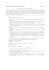More information

### The Essential Spectra of Toeplitz Operators with Symbols in Hâ + C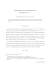More information

### ax + by ≡ 1 MODULO xy ON THE CONGRUENCE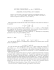More information

### Full textMore information

### Document 6529312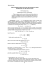More information

### Document 6529918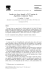More information

### Probabilistic low-rank matrix completion on finite alphabets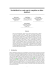More information

### 91.304 Foundations of Computer Science Fall 2010 Sample Midterm Quiz HintMore information

### Media Kit - Urban CuspMore information

### Lecture 12: Randomized Rounding Algorithms forMore information

### annates acadeii{iae scientiarum fennicae boundary values and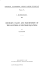can be used to solve Dirichlet,'s problem in t'he plane. Our method is based on the general principle that if we know the solution of the boundary value problem in the small, we get a solution in t...

More information

### a characterization of finitely monotonic additive functionMore information

### ASYMPTOTIC DISTRIBUTION OF THE MAXIMUM VECTORS WITH A SPHERICALLY SYMMETRIC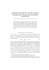More information

### Statistics and Probability Letters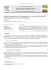More information

### SOME SAMPLE FUNCTION PROPERTIES OF A PROCESS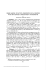More information

### What mathematics is hidden behind the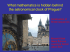More information

### Document 6511833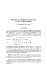More information

### Document 6527827More information

### Midterm II Information and Review - Full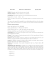More information

### EXISTENCE AND UNIQUENESS FOR A QUASILINEAR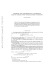More information

### Factorization for Formal Laurent Series and Lattice Path Enumeration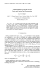More information

### BACKWARD UNIQUENESS FOR PARABOLIC EQUATIONS LMore information